## SIMULATION OF BEHAVIOUR OF TYPICAL CONCRETE SPECIMEMS BASED ON A NONLOCAL MACRO-MESO-SCALE CONSISTENT DAMAGE MODEL1)

Ren Yudong, Chen Jianbing,2)

College of Civil Engineering & State Key Laboratory of Disaster Reduction in Civil Engineering, Tongji University, Shanghai 200092, China

 基金资助: 1)国家杰出青年科学基金.  51725804

Received: 2020-12-13   Accepted: 2021-03-5   Online: 2021-04-08Abstract

Concrete is a typical quasi-brittle material, and the nonlinear analysis and crack simulation of concrete during loading are still challenging issues. Classical fracture mechanics and damage mechanics describe crack topology from discrete and continuous perspectives respectively, and became two of the most powerful tools for solid crack simulation and prediction problems. Since the beginning of this century, in the phase field theory and peridynamics significant progress has been made in predicting the crack initiation and propagation and nonlinear analysis. Recently, a new nonlocal macro-meso-scale consistent damage (NMMD) model has been developed based on the basic ideas of phase field theory and peridynamics. In this model, the concepts of material point pair are introduced to characterize the meso-scale damage due to deformation. Then the topologic damage which quantifies the degree of discontinuity in macroscopic solid is defined as the weighted average of meso-scale damage in the influence domain. Through the physically-based energetic degradation function which bridges the topologic damage and energy dissipation, the topologic damage can be inserted into the framework of continuum damage mechanics, which allows this model to simulate the crack process naturally while performing nonlinear analysis without prescribed initial crack and potential propagation path. The present paper takes into account the spatial variability of the meso-scale physical parameters and employs the NMMD model to simulate the whole loading process of typical concrete specimens. The model meso-scale parameters are calibrated through the 1D modeling firstly, and the relationship between the meso-scale parameters and the meso-scale physical-geometric properties of concrete is discussed. Based on the 1D-calibrated parameters, a detailed analysis through the 2D NMMD model is performed. Further, the influence of material parameter spatial variability on the behaviors of uniaxial tensile concrete specimen and notched three-point bending beam is investigated. The work in this paper provides a meaningful reference for the calibration of meso-scale parameters in the NMMD model and the investigation on nonlinear mechanical behavior of concrete and other quasi-brittle materials under complex stress state.

Keywords： concrete ; nonlocal macro-meso-scale consistent damage model ; uniaxial tension ; parameter calibration ; parameter spatial variability

Ren Yudong, Chen Jianbing. SIMULATION OF BEHAVIOUR OF TYPICAL CONCRETE SPECIMEMS BASED ON A NONLOCAL MACRO-MESO-SCALE CONSISTENT DAMAGE MODEL1). Chinese Journal of Theoretical and Applied Mechanics[J], 2021, 53(4): 1196-1121 DOI:10.6052/0459-1879-20-427

## 引言

21世纪初以来, 混凝土本构关系的研究在两个方面同时取得了新的重要进展. 一方面, 由于试验技术的进步, 已经能够获得混凝土单轴受压应力-应变全曲线, 甚至可获得混凝土单轴受拉全曲线.[9-10]. 另一方面, 混凝土损伤力学的发展有效地促进了混凝土本构关系从经验为主向理性建模的转变进程. 在这一进程中, 建立合理反映具有多相复合特征和准脆性性质的混凝土受力行为的微细观物理机制、从而把握裂纹萌生与发展过程具有重要意义.

20世纪20年代以来, 断裂力学[11-15]和损伤力学[1,16-18]得到了极大的发展. 两者分别从不连续和连续的角度对裂纹进行描述, 形成了固体破坏问题研究的两大分支. 在计算方面, 为了描述裂纹引起的不连续性, 发展了内聚裂缝单元、扩展有限元法与无网格法等一系列数值算法. 21世纪初, Bourdin等[22-23]开创性地将断裂力学与损伤力学结合起来, 发展了力学中的相场理论, 得到了大量关注与应用[24-26]. 近年来, Wu等[27-28]将相场理论与内聚裂缝模型结合起来, 发展了统一相场理论, 成功地解决了一系列脆性与准脆性材料的静力与动力破坏分析问题[29-32]. 同样在21世纪初, Silling[33-36]提出了近场动力学方法(也称毗域动力学), 在模拟材料损伤破坏、裂纹扩展方面取得了重要进展[38-39]. 最近, Lu和Chen[40-41]结合统一相场理论和近场动力学的基本思想, 提出了一类非局部宏-微观损伤模型, 为准脆性材料的破坏问题分析提供了新的视角. 非局部宏-微观损伤模型引入近场动力学中物质点和物质点偶的概念来刻画由于变形引起的微细观损伤, 进而以作用域内的微细观损伤之加权平均来度量物质不连续程度, 即宏观拓扑损伤, 从而对损伤何时发生、损伤如何演化的问题做出了回答. 通过能量退化函数, 将拓扑损伤嵌入到连续介质-损伤力学的框架中. 在此基础上, Chen等基于能量等效的基本思想对能量退化函数进行了物理建模, 给出了宏-微观损伤模型中能量退化函数的理性表达.

## 1 连续介质损伤力学基本框架

### 1.1 本构方程

$\begin{eqnarray} \label{eq1} \psi =g\psi_{0} \end{eqnarray}$

$\begin{eqnarray} \label{eq2} \psi_{0} =\frac{1}{2}{\epsilon }:{D}:{\epsilon } \end{eqnarray}$

$\begin{eqnarray} \label{eq3} \varXi ={\sigma }:{\dot{{\epsilon }}}-\dot{{\psi }}\geqslant 0 \end{eqnarray}$

$\begin{eqnarray} \label{eq4} \left( {{\sigma }-g{D}:{\epsilon }} \right):{\dot{{\epsilon }}}-\dot{{g}}\psi_{0} \geqslant 0 \end{eqnarray}$

$\begin{eqnarray} \label{eq5} {\sigma }=g{D}:{\epsilon } \end{eqnarray}$

$\begin{eqnarray} \label{eq6} -\dot{{g}}\psi_{0} \geqslant 0 \end{eqnarray}$

### 1.2 平衡方程与边界条件

$\begin{eqnarray} \label{eq7} \int_{\cal B} {{b}{d}V} +\oint_{\partial {\cal B}} {{t}{d}S} ={\bf 0} \end{eqnarray}$

$\begin{eqnarray} \label{eq8} \mbox{div}\left( {{\sigma }} \right)+{b}={\bf0},\ \ \forall {x}\in {\cal B} \end{eqnarray}$

$\begin{eqnarray} \label{eq9} \left. {\begin{array}{l} {u}={\bar{{u}}},\ \ \forall {x}\in \partial_{u} {\cal B} \\ {\sigma }\cdot {\eta }_{{t}} ={\bar{{t}}},\ \ \forall {x}\in \partial_{t} {\cal B} \\ \end{array}} \right\} \end{eqnarray}$

$\begin{eqnarray} \label{eq10} \partial_{u} {\cal B}\cup \partial_{t} {\cal B}=\partial {\cal B},\ \ \ \partial _{u} {\cal B}\cap \partial_{t} {\cal B}=\emptyset \end{eqnarray}$

## 2 非局部宏-微观损伤模型

### 2.1 微细观损伤与拓扑损伤

$\begin{eqnarray} \label{eq11} \lambda^{+}\left( {{x},{x'},t} \right)=\left\langle {\left\| {{\xi }+{\eta }} \right\|_{2} -\left\| {{\xi }} \right\|_{2} } \right\rangle \end{eqnarray}$

$\begin{eqnarray} \label{eq12} \kappa \left( {{x},{x'},t} \right)=\mathop{\mbox{max}}\limits_{\tau \in \left[ {0,t} \right]} \left\langle {\lambda^{+}\left( {{x},{x'},\tau } \right)-\lambda_{c} } \right\rangle \end{eqnarray}$

$\begin{eqnarray} \label{eq13} \omega \left( {{x},{x'},t} \right)=1-\exp \left[ {-\gamma \kappa \left( {{x},{x'},t} \right)} \right],\ \ \gamma >0 \end{eqnarray}$

$\begin{eqnarray} \label{eq14} {\cal D}_{\ell } \left( {{x}} \right)=\left\{ {{x'}\vert \left\| {{x'}-{x}} \right\|_{2} <\ell ,\ \ {x'}\in {\cal B}} \right\} \end{eqnarray}$

$\begin{eqnarray} \label{eq15} \varOmega \left( {{x},t} \right)=\int_{{\cal D}_{\ell } ({x})} {\varphi \left( {{x},{x'}} \right)\omega \left( {{x},{x'},t} \right){d}{V}'} \end{eqnarray}$

$\begin{eqnarray} \label{eq16} \varphi \left( {{x},{x'}} \right)=\left\{ {{\begin{array}{ll} \dfrac{5}{4\ell }\left( {1+3R} \right)\left( {1-R} \right)^{3},\ \ & n=1 \\[3mm] \dfrac{5}{\pi \ell^{2}}\left( {1+3R} \right)\left( {1-R} \right)^{3},\ \ &n=2 \\ \end{array} }} \right. \end{eqnarray}$

### 2.2 能量退化函数及其物理建模

$\begin{eqnarray} \label{eq17} \left. {\begin{array}{l} g=t^{3} \\ \varOmega =-\dfrac{3}{2}\left( {1-t} \right)^{3}+\dfrac{5}{2}\left( {1-t} \right)^{5} \\ \end{array}} \right\} \end{eqnarray}$

$\begin{eqnarray} \label{eq18} \left( {1-\varOmega } \right)^{\alpha }+\left( {1-g} \right)^{\alpha }=1 \end{eqnarray}$

$\begin{eqnarray} \label{eq19} g\left( \varOmega \right)=1-\left[ {1-\left( {1-\varOmega } \right)^{\alpha }} \right]^{1/\alpha } \end{eqnarray}$

## 3 单轴受拉板式试件力学行为模拟

### 图1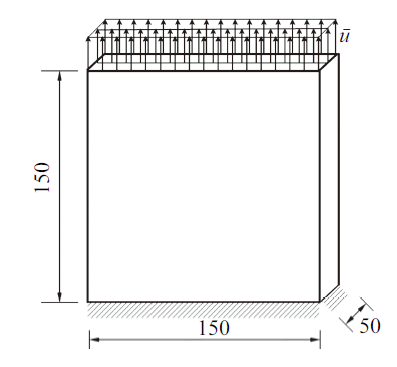Fig.1   Schematic diagram of uniaxial tension panel specimen

### 图2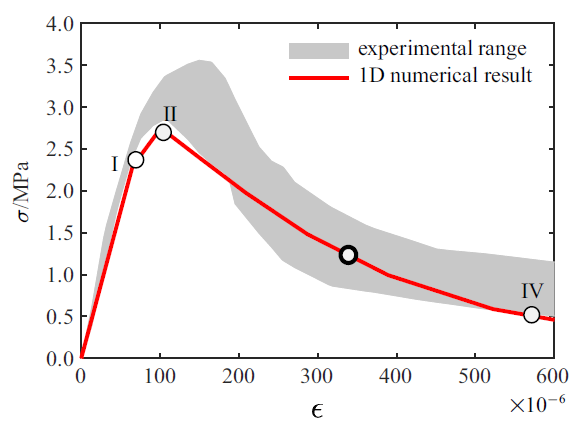Fig.2   Stress versus strain curve calculated from the 1D model

### 图3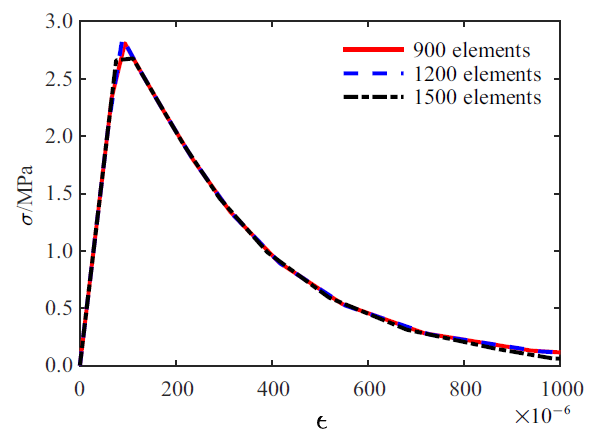Fig.3   Stress versus strain curves from different meshes in 1D model

### 图4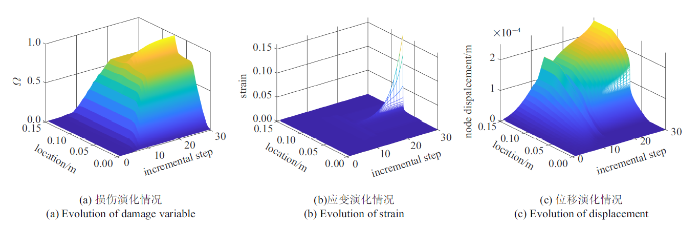### 图5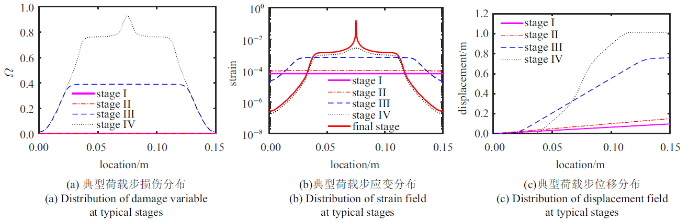Fig.5   Distribution of field variables at typical load steps (1500 elements)

### 图6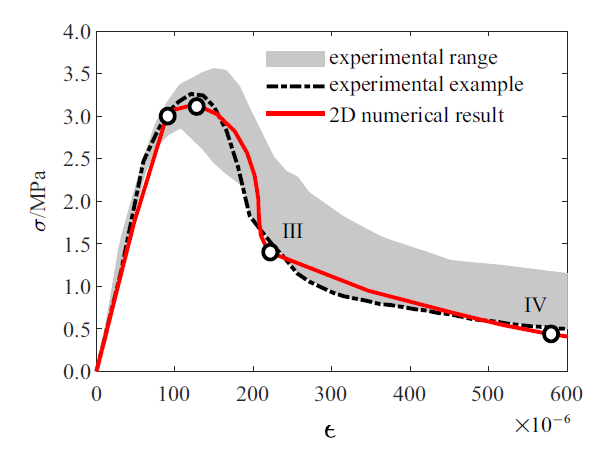Fig.6   Stress versus strain curve calculated from 2D model

### 图7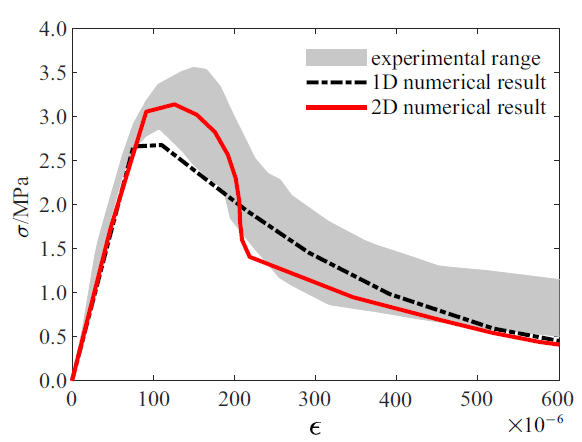Fig.7   Stress versus strain curves from 1D model, 2D model and experiment

### 图8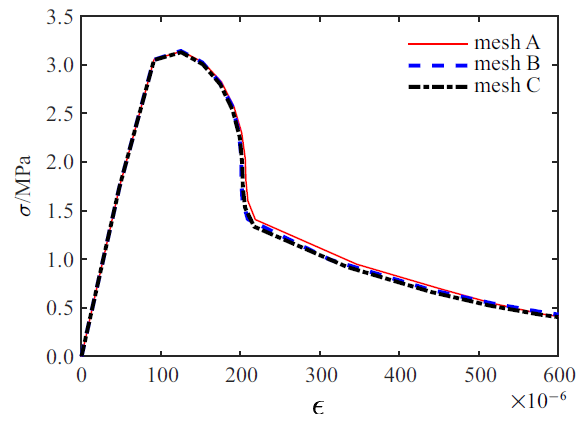Fig.8   Stress versus stress curves from different meshes in 2D model

### 图9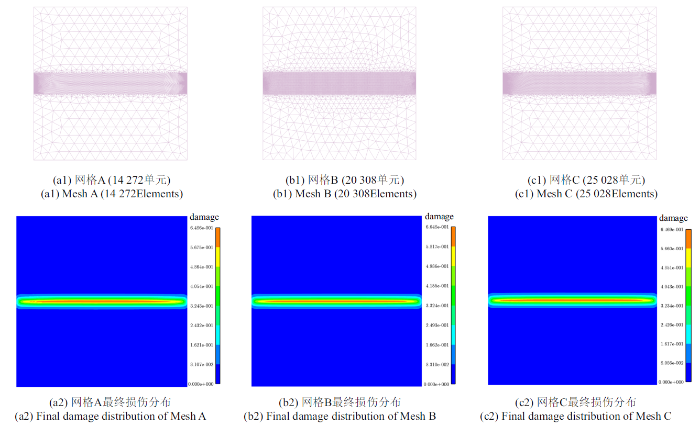Fig.9   Finite element discretization and damage distribution in the final stage

### 图10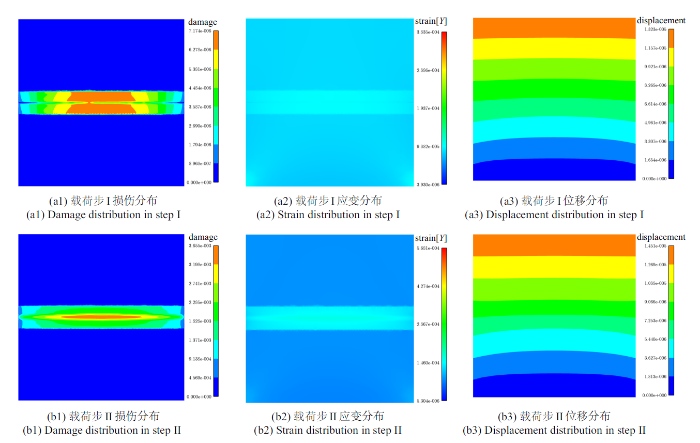Fig.10   Distribution of field variables in 2D model at typical load steps (Mesh C)

### 图11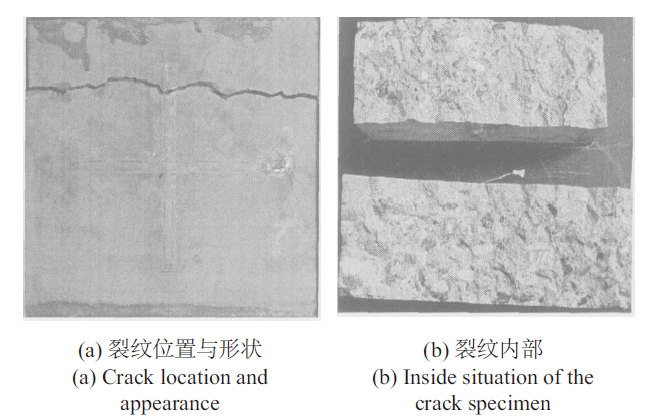Fig.11   Crack location and appearance of typical specimen

### 图12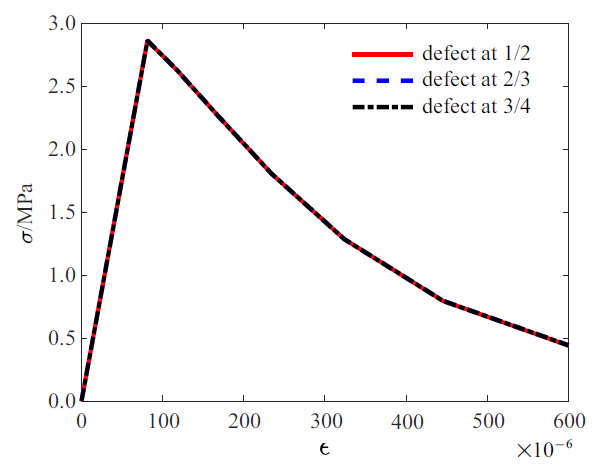Fig.12   The stress versus strain curves from 1D model with different defect locations

### 图13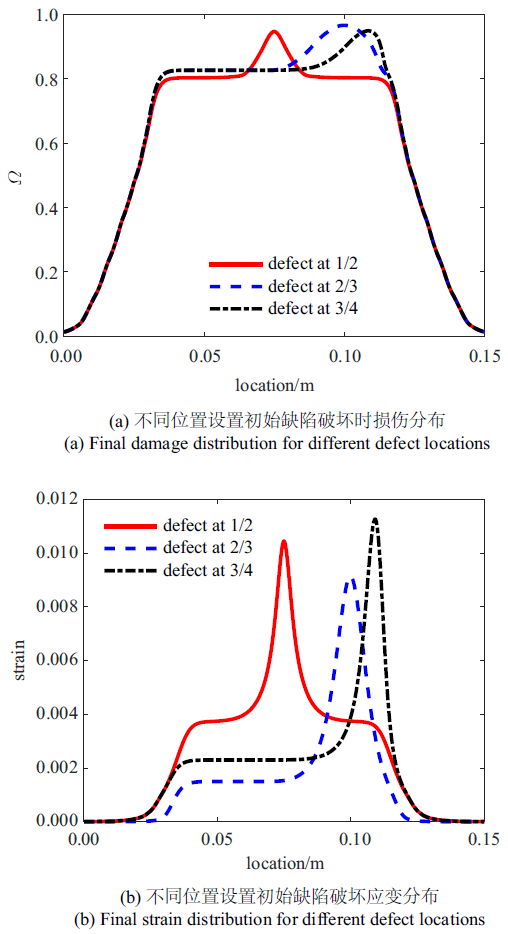Fig.13   Final field variables distributions of 1D model with different defect locations

## 4 考虑参数空间变异性的混凝土力学行为模拟

### 图14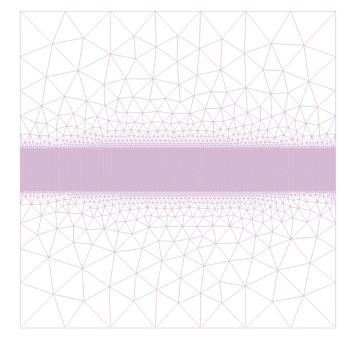Fig.14   Mapped mesh (26 910 elements, 24 000 elements in the encryption region)

$\begin{eqnarray} \label{eq20} S\left( {\kappa_{1} ,\kappa_{2} } \right)=\frac{\sigma^{2}a^{2}}{4\pi}\exp \left[ {\frac{a^{2}}{4}\left( {\kappa_{1}^{2} +\kappa_{2}^{2} } \right)} \right] \end{eqnarray}$

### 图15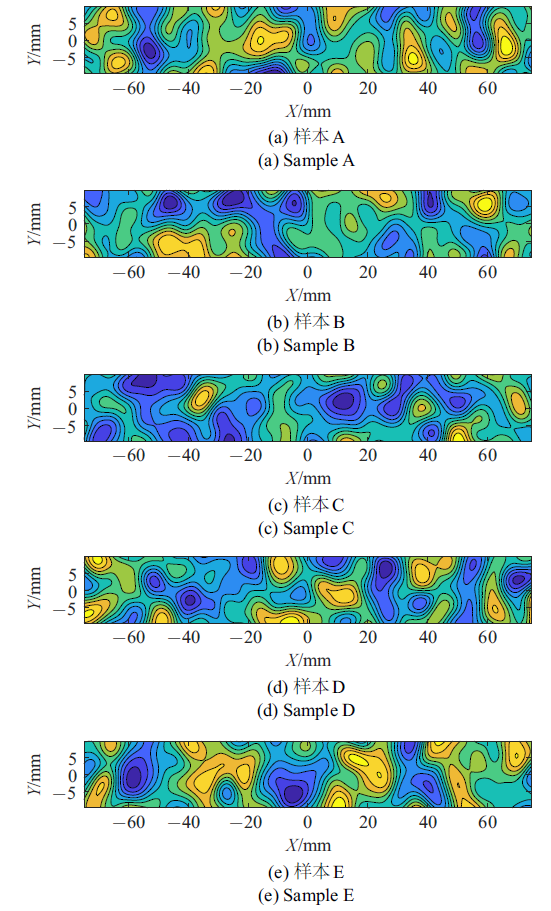Fig.15   Samples of critical elongation quantity random field

### 图16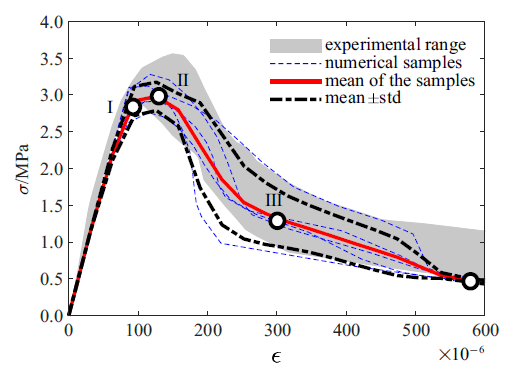Fig.16   Whole strain-stress curve samples and the mean value curve with the standard deviation curve obtained after considering the critical elongation quantity as a random field

### 图17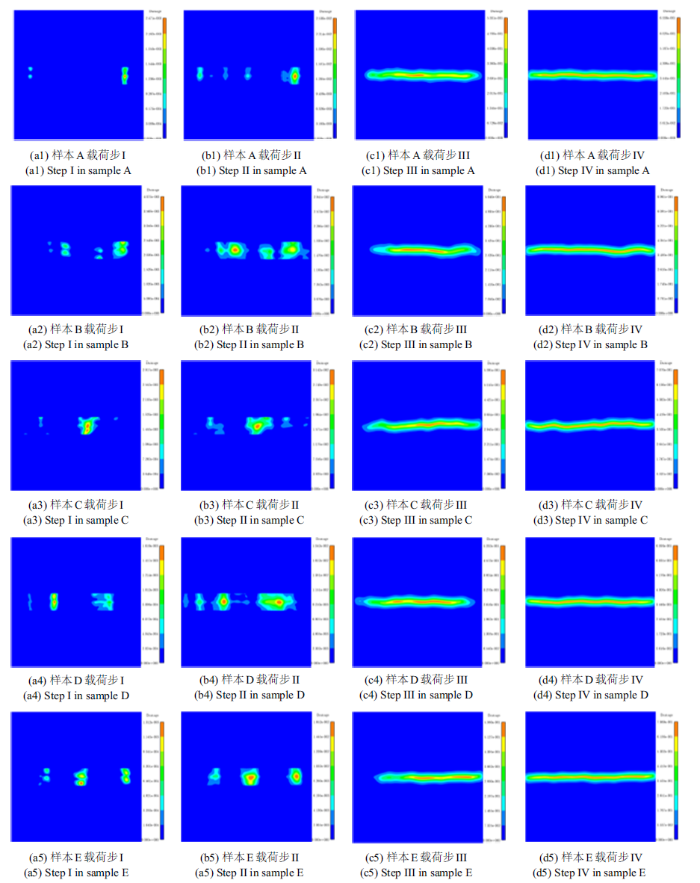Fig.17   Crack propagation process of different samples after considering the critical elongation quantity as random field

### 图18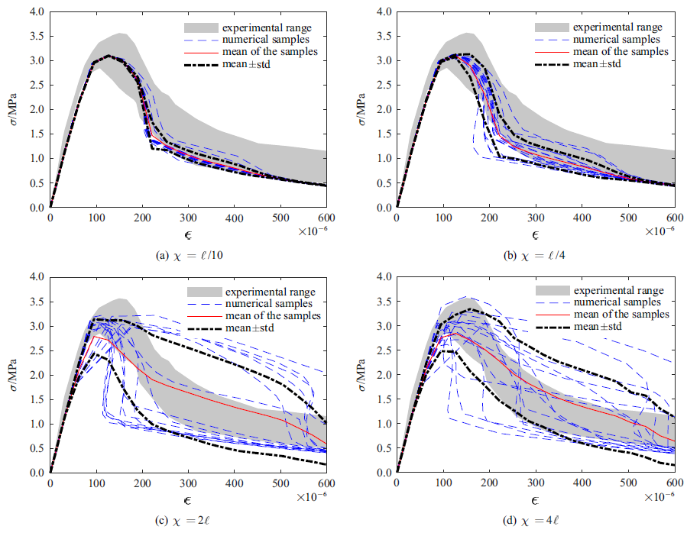Fig.18   Influence of correlation length of parameter random field on calculation results

### 图19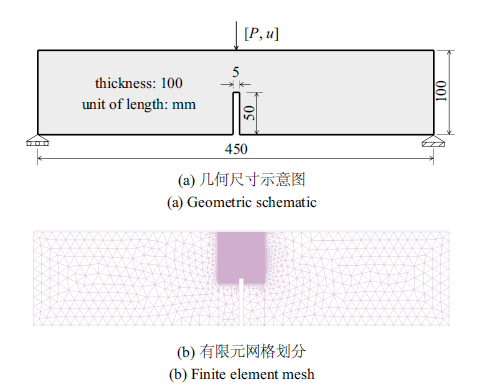Fig.19   Geometric schematic and finite element meshes of the notched beam

### 图20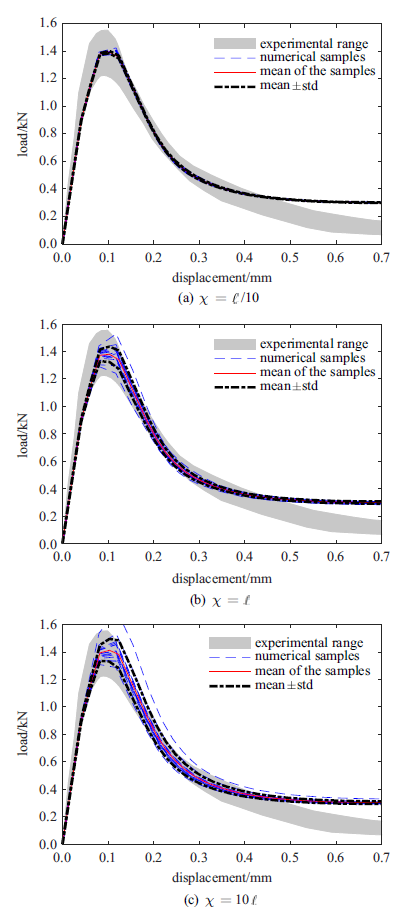Fig.20   Load-deformation curve samples and the mean value curve with the standard deviation curves of the three point bending notched beam

## 5 结论

(1)利用能量等效的思想对一维情况的能量退化函数进行了物理建模并给出其参数方程形式的解析表达. 利用星形线对一维、二维能量退化函数进行拟合, 给出了显式近似表达, 便于实际应用.

(2)首先利用一维非局部宏-微观损伤模型基于试验标定参数, 进而采用二维宏-微观损伤模型进行精细化分析, 显著降低了标定参数的时间成本. 同时, 分析了宏-微观损伤模型中模型参数与混凝土材料的细观物理-几何特性之间的联系, 可为模型细观参数的物理意义及定量标定提供指导.

(3)考察了细观参数空间变异性对混凝土单轴受拉试件和带缺口三点弯曲试件两类受力性质不同的试件力学行为的影响. 分析结果表明非局部宏-微观损伤模型不仅可以合理地反映非线性的发展, 还可以结合参数随机场把握混凝土内禀的随机性. 此时毋须预设微小缺陷, 且裂纹自发萌生与发展, 更符合实际情况. 计算结果还表明, 当参数随机场的相关长度逐渐减小时, 应力-应变全曲线的变异性随之降低. 单轴受拉与三点受弯缺口梁试件的对比分析则进一步表明, 当裂纹扩展路径的随机性更大时, 载荷-变形曲线的变异性随之增大.

## 参考文献 原文顺序 文献年度倒序 文中引用次数倒序 被引期刊影响因子

(Li Jie, Wu Jianying, Chen Jianbing. Stochastic Damage Mechanics of Concrete Structures. Beijing: Science Press, 2014 (in Chinese))

( General Administration of Quality Supervision, Inspection and Quarantine of the People's Republic of China, Standardization Administration of the People's Republic of China. Seismic Ground Motion Parameters Zonation Map of China//GB18306-2015. Beijing: Standards Press of China, 2015 (in Chinese))

(Yang Muqiu, Lin Hong.

Experimental study on whole stress-strain under uniaxial compression and tension

Journal of Hydraulic Engineering, 1992(6):60-66 (in Chinese))

Yue JG, Kunnath SK, Xiao Y.

Uniaxial concrete tension damage evolution using acoustic emission monitoring

Construction and Building Materials, 2020,232:117281

(Li Jie, Lu Zhaohui, Zhang Qiyun.

Study on stochastic damage constitutive law for concrete material subjected to uniaxial compressive stress

Journal of Tongji University, 2003,31(5):505-509 (in Chinese))

(Liu Hankun, Li Jie.

Nonlocal micro-damage model for concrete under uniaxial tension

Journal of Tongji University, 2014,42(2):203-209 (in Chinese))

(Wu Xiang, Li Jie.

Meso-mechanism based simulation of concrete damage process

Journal of Building Materials, 2009,12(3):259-265 (in Chinese))

(Li Jie, Yang Weizhong.

Elastoplastic stochastic damage constitutive law for concrete

China Civil Engineering Journal, 2009,42(2):31-38 (in Chinese))

[硕士论文]. 上海: 同济大学, 2006

(Ren Xiaodan.

Experimental research on stochastic damage constitutive law of concrete

[Master Thesis]. Shanghai: Tongji University, 2006 (in Chinese))

[硕士论文]. 湖南: 湖南大学, 2006

(Wu Feng.

Experimental study on whole stress-strain curves of concrete under axial tension

[Master Thesis]. Changsha: Hunan University, 2006 (in Chinese))

Griffith AA.

The phenomena of flow and rupture in solids

Philosophical Transactions of The Royal Society, 1920,221:163-198

Irwin GR.

Analysis of stresses and strains near the end of a crack transversing a plate

Journal of Applied Mechanics, 1957,24:361-364

Rice JR.

A path independent integral and the approximate analysis of strain concentration by notches and cracks

Journal of Applied Mechanics, 1968,35(2):379-386

Hutchinson JW.

Singular behaviour at the end of a tensile crack in a hardening material

Journal of the Mechanics and Physics of Solids, 1968,16(1):13-31

Rice JR, Rosengren GF.

Plane strain deformation near a crack tip in a power-law hardening material

Journal of the Mechanics and Physics of Solids, 1968,16(1):1-12

Kachanov LM.

On the rupture time under the condition of creep

Izv. Akad. Nauk, Otd. Tekh. Nauk, 1958,8:26-31

Ju JW.

On energy-based coupled elastoplastic damage theories: Constitutive modeling and computational aspects

International Journal of Solids and Structures, 1989,25(7):803-833

Wu JY, Li J, Faria R.

An energy release rate-based plastic-damage model for concrete

International Journal of Solids and Structures, 2006,43(3-4):583-612

Carpinteri A, Cornetti P, Barpi F, et al.

Cohesive crack model description of ductile to brittle size-scale transition: Dimensional analysis vs. renormalization group theory

Engineering Fracture Mechanics, 2003,70(14):1809-1839

Belytschko T, Black T.

Elastic crack growth in finite elements with minimal remeshing

International Journal for Numerical Methods in Engineering, 1999,45(5):601-620

Chen JS, Hillman M, Chi SW.

Meshfree methods: Progress made after 20 years

Journal of Engineering Mechanics, 2017,143(4):04017001

Bourdin B, Francfort GA, Marigo JJ.

Numerical experiments in revisited brittle fracture

Journal of the Mechanics and Physics of Solids, 2000,48(4):797-826

Bourdin B, Francfort GA, Marigo JJ.

The variational approach to fracture

Journal of Elasticity, 2008,91(1-3):5-148

Miehe C, Welschinger F, Hofacker M.

Thermodynamically consistent phase-field models of fracture: Variational principles and multi-field FE implementations

International Journal for Numerical Methods in Engineering, 2010,83(10):1273-1311

Miehe C, Hofacker M, Welschinger F.

A phase field model for rate-independent crack propagation: robust algorithmic implementation based on operator splits

Computer Methods in Applied Mechanics and Engineering, 2010,199(45):2765-2778

Borden MJ, Hughes TJR, Landis CM, et al.

A higher-order phase-field model for brittle fracture: Formulation and analysis within the isogeometric analysis framework

Computer Methods in Applied Mechanics and Engineering, 2014,273:100-118

Wu JY.

A unified phase-field theory for the mechanics of damage and quasi-brittle failure

Journal of the Mechanics and Physics of Solids, 2017,103:72-99

Wu JY, Nguyen VP.

A length scale insensitive phase-field damage model for brittle fracture

Journal of the Mechanics and Physics of Solids, 2018,119:20-42

Wu JY.

A geometrically regularized gradient-damage model with energetic equivalence

Computer Methods in Applied Mechanics and Engineering, 2018,328:612-637

(Wu Jianying.

On the theoretical and numerical aspects of the unified phase-field theoryfor damage and failure in solids and structures

Chinese Journal of Theoretical and Applied Mechanics, 2021,53(2):301-329 (in Chinese))

Hai L, Wu JY, Li J.

A phase-field damage model with micro inertia effect for the dynamic fracture of quasi-brittle solids

Engineering Fracture Mechanics, 2020,225:106821

(Wu Jianying, Chen Wanxin, Huang Yuli.

Computational modeling of shrinkage induced cracking in early-age concrete based on the unified phase-field theory

Chinese Journal of Theoretical and Applied Mechanics 2021 (in Chinese))

Silling SA.

Reformulation of elasticity theory for discontinuities and long-range forces

Journal of the Mechanics and Physics of Solids, 2000,48(1):175-209

A meshfree method based on the peridynamic model of solid mechanics

Computers & Structures, 2005,83(17):1526-1535

Silling SA, Epton M, Weckner O, et al.

Peridynamic states and constitutive modeling

Journal of Elasticity, 2007,88(2):151-184

(Huang Dan, Zhang Qing, Qiao Peizhong, et al.

A review on peridynamics (PD) method and its applications

Advances in Mechanics, 2010,40(4):448-459 (in Chinese))

(Du Qiang.

From peridynamics to stochastic jump process: Illustrations of nonlocal balance laws and nonlocal calculus framework

Science China Mathematics, 2015,45(7):939-952 (in Chinese))

Huang D, Lu GD, Qiao PZ.

An improved peridynamic approach for quasi-static elastic deformation and brittle fracture analysis

International Journal of Mechanical Sciences, 2015, 94-95:111-122

Gerstle W, Sau N, Silling S.

Peridynamic modeling of concrete structures

Nuclear Engineering and Design, 2007,237(12):1250-1258

Lu GD, Chen JB.

A new nonlocal macro-meso-scale consistent damage model for crack modeling of quasi-brittle materials

Computer Methods in Applied Mechanics and Engineering, 2020,362:112802

( Lu Guangda, Chen Jianbing.

Cracking simulation based on a nonlocal macro-meso-scale damage model

Chinese Journal of Theoretical and Applied Mechanics, 2020,52(3):1-14 (in Chinese))

Chen JB, Ren YD, Lu GD.

Meso-scale physical modeling of energetic degradation function in the nonlocal macro-meso-scale consistent damage model for quasi-brittle materials

Computer Methods in Applied Mechanics and Engineering, 2021,374:113588

May IM, Duan Y.

A local arc-length procedure for strain softening

Computers & Structures, 1997,64(1):297-303

Influence of the roughness of aggregate surface on the interface bond strength

Cement and Concrete Research, 2002,32(2):253-257

Vanmarcke E.

Random Fields: Analysis and Synthesis

Singapore: World Scientific, 2010

Chen JB, He JR, Ren XD, et al.

Stochastic harmonic function representation of random fields for material properties of structures

Journal of Engineering Mechanics, American Society of Civil Engineers, 2018,144(7):04018049

(Liang Shixue, Ren Xiaodan, Li Jie.

A random medium model for simulation of concrete failure

Sci China Tech Sci, 2013,56:1273-1281 (in Chinese))

/

 〈〉